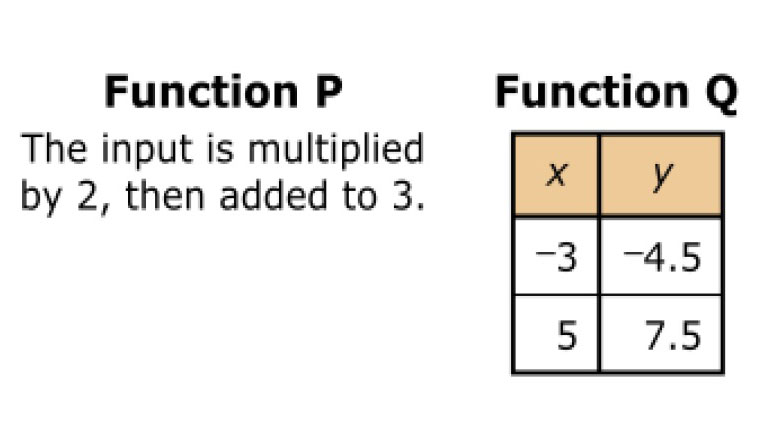Friday, September 17, 2021# Grade 8 PARCC math: functions as rules

-

#### The following inline choice question, explained here in hopes of helping eighth-grade students and their parents in Maryland and Illinois prepare for the PARCC test near the end of this school year, appears on the released version of PARCC’s Spring 2016 test for grade eight math:Information about two linear functions is shown.Select from the drop-down menus to correctly complete each sentence.

The average rate of change of function P is __A__ the average rate of change of function Q.

The y-intercept of function P is __B__ the y-intercept of function Q.

### Choices for both _A_ and _B_:

• less than       • equal to       • greater than

Correct answer: P’s rate of change is greater than Q’s, and P’s y-intercept is greater than Q’s.

Common Core Math Content 8th grade, Functions, Define, evaluate, and compare functions.

(8.F.A.2) Compare properties of two functions each represented in a different way (algebraically, graphically, numerically in tables, or by verbal descriptions). For example, given a linear function represented by a table of values and a linear function represented by an algebraic expression, determine which function has the greater rate of change.

The ability to compare properties of functions like this also depends on a few other part of the Common Core, including the following:

(8.F.A.1) Understand that a function is a rule that assigns to each input exactly one output. The graph of a function is the set of ordered pairs consisting of an input and the corresponding output.

(8.F.A.3) Interpret the equation y = mx + b as defining a linear function, whose graph is a straight line; give examples of functions that are not linear. For example, the function A = s2 giving the area of a square as a function of its side length is not linear because its graph contains the points (1,1), (2,4) and (3,9), which are not on a straight line.

And even (8.F.B.4) Construct a function to model a linear relationship between two quantities. Determine the rate of change and initial value of the function from a description of a relationship or from two (x, y) values, including reading these from a table or from a graph. Interpret the rate of change and initial value of a linear function in terms of the situation it models, and in terms of its graph or a table of values.

Solution strategy (there are others)

Find the slope and intercept of function P and function Q.

Function P is defined by a rule. We can translate this rule directly into an equation that describes a linear function:$y = 2x + 3$

Since that is in the slope-intercept form for the equation of a line, y = mx + b, the slope is 2, which also represents the rate of change, and the y-intercept is 3.

For function Q, we have to compute the rate of change, which is given by$\textrm{slope} = \frac{\Delta y}{\Delta x} = \frac{y_2 - y_1}{x_2 - x_1}$

The change in y, 7.5 – (–4.5), is 12, and the change in x, 5 – (–3), is 8, giving us a rate of change of 1.5.

Where does function Q cross the y-axis? For this, we can plug in one of the points that we know is on the line for function Q and find the y-intercept:$7.5 = (1.5)(5) + b$$0 = b$

## Analysis of this question and online accessibility

Eighth-grade math students have to grasp the concept of a function as a rule that assigns to each input exactly one output. Functions describe situations where one quantity determines another. This skill and the understanding of the underlying notion of a function is mostly new in eighth grade, even though this understanding has significant prerequisite understanding that is part of the sixth- and seventh-grade Common Core standards.

In eighth grade, students learn to translate among representations and partial representations of functions, noting that rule-based, tabular, and graphical representations may be partial representations. They should be able describe how aspects of the function are reflected in the different representations.

The question can be delivered online and on paper-based tests, and the validity, reliability, and fairness measures should not differ significantly among the various delivery modes.

The use of the adjective “average” to describe the rate of change of a function that a test-taker knows is linear (because the problem identifies both P and Q as linear functions) is at best redundant and at worst misleading to eighth-graders who may incorrectly think, for a moment, that the rate of change for a linear function itself is subject to change and an “average” value must somehow be computed. The word isn’t mathematically incorrect, however, and its use simply makes the problem less direct, not invalid.

Still, the use of the word average does force our analysis to wonder whether this word is a Freudian-like slip or a canary in the mine that reveals for us all an understanding of linear functions on the part of the people who developed this test question that is essentially below the eighth-grade level. If, on the other hand, the word is used intentionally, it is misleading.

• Khan Academy finds “average” rate of change for linear and nonlinear functions

No special accommodation challenges can be identified with this question, so the question is considered fair.Paul Katulahttps://news.schoolsdo.org
Paul Katula is the executive editor of the Voxitatis Research Foundation, which publishes this blog. For more information, see the About page.

### Study finds increasing suicide rates among Black girls

0
Why are Black adolescent girls committing suicide at increasingly higher rates in the US? Could it be an effect of racism and prejudice?